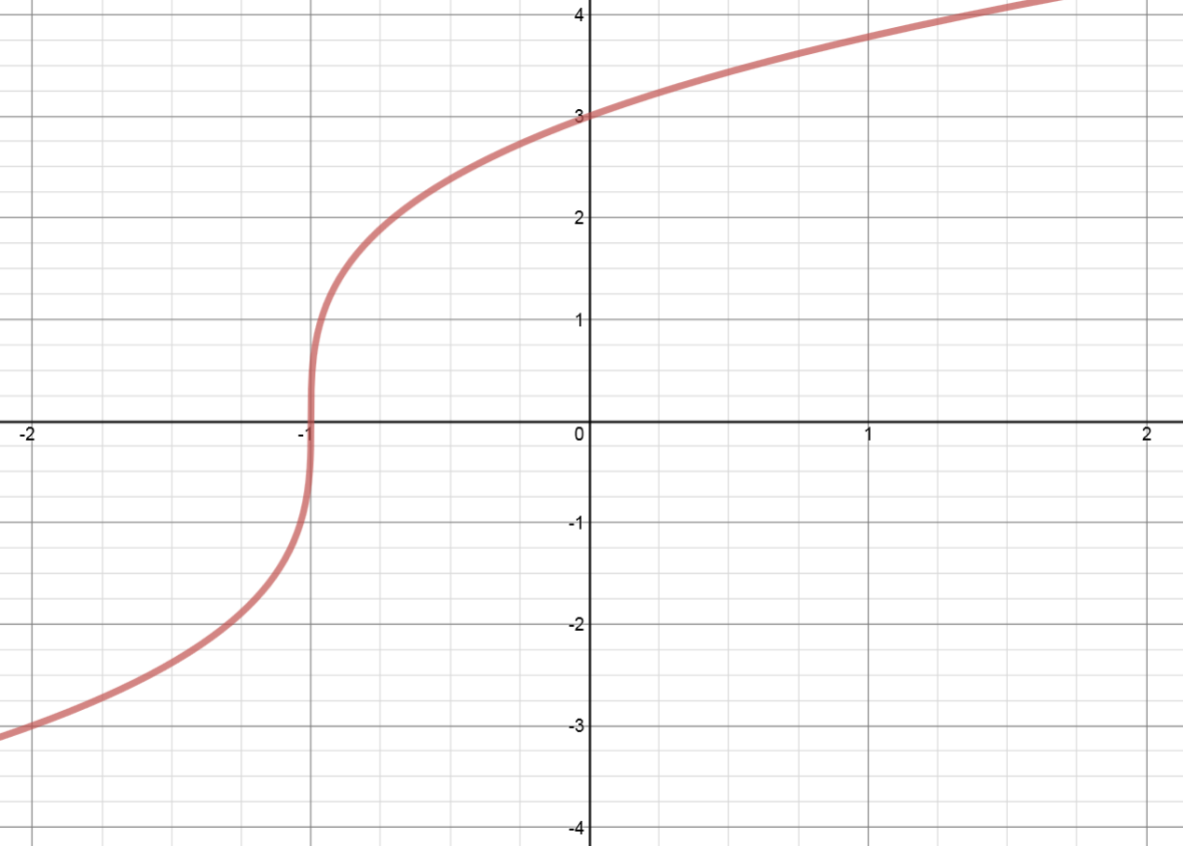# Reflection across the y-axis: $y = f(-x)$

Before we get into reflections across the y axis, make sure you've refreshed your memory on how to do simple vertical translation and horizontal translation.

## Reflection Across the Y-Axis

One of the most basic transformations you can make with simple functions is to reflect it across the y-axis or another vertical axis. In a potential test question, this can be phrased in many different ways, so make sure you recognize the following terms as just another way of saying "perform a reflection across the y-axis":

• Graph $y = f(-x)$

• Graph $f(-x)$

$f(-x)$ reflection

• Or simply: $f(-x)$

In order to do this, the process is extremely simple: For any function, no matter how complicated it is, simply pick out easy-to-determine coordinates, divide the x-coordinate by (-1), and then re-plot those coordinates. That's it!

The best way to practice drawing reflections over y axis is to do an example problem:

Example:

Given the graph of $y=f(x)$ as shown, sketch $y = f(-x)$.

Remember, the only step we have to do before plotting the f(-x) reflection is simply divide the x-coordinates of easy-to-determine points on our graph above by (-1). When we say "easy-to-determine points" what this refers to is just points for which you know the x and y values exactly. Don't pick points where you need to estimate values, as this makes the problem unnecessarily hard. Below are several images to help you visualize how to solve this problem.

Step 1: Know that we're reflecting across the y-axis

Step 2: Identify easy-to-determine points

Step 3: Divide these points by (-1) and plot the new points

## How to Find the Axis of Symmetry

In some cases, you will be asked to perform vertical reflections across an axis of symmetry that isn't the y-axis. But before we go into how to solve this, it's important to know what we mean by "axis of symmetry". The axis of symmetry is simply the vertical line that we are performing the reflection across. It can be the y-axis, or any vertical line with the equation x = constant, like x = 2, x = -16, etc.

Finding the axis of symmetry, like plotting the reflections themselves, is also a simple process. In this case, all we have to do is pick the same point on both the function and its reflection, count the distance between them, and divide that by 2. This is because, by it's definition, an axis of symmetry is exactly in the middle of the function and its reflection.

The best way to practice finding the axis of symmetry is to do an example problem:

Example:

Find the axis of symmetry for the two functions show in the image below.

Again, all we need to do to solve this problem is to pick the same point on both functions, count the distance between them, and divide by 2. Let's pick the origin point for these functions, as it is the easiest point to deal with.

Now, by counting the distance between these two points, you should get the answer of 8 units. The last step is to divide this value by 2, giving us x = 4 as our axis of symmetry! Let's take a look at what this would look like if there were an actual line there:

And that's all there is to it! For further study with transformations of a functions with regards to trigonometric functions, see our lessons on transformations of trig graphs and how to find trigonometric functions by graphs.

### Reflection across the y-axis: $y = f(-x)$

Besides translations, another kind of transformation of function is called reflection. If a reflection is about the y-axis, then, the points on the right side of the y-axis gets to the right side of the y-axis, and vice versa.

#### Lessons

• Introduction
An Experiment to Study "Reflection Across the Y-axis"
Sketch and compare: $y = {\left( {x - 4} \right)^3}$      VS.      $y = {\left( { - x - 4} \right)^3}$
a)
Sketch both cubic functions on the same set of coordinate axes.

b)
Compared to the graph of $y = {\left( {x - 4} \right)^3}$:
• the graph of $y = {\left( { - x - 4} \right)^3}$ is a reflection in the ________________________.

• 1.
Reflection Across the Y-axis
Given the graph of $y = f\left( x \right)$ as shown, sketch:
a)
$y = f\left( { - x} \right)$

b)
In conclusion:
$\left( x \right) \to \left( { - x} \right)$: reflection in the _________________________ $\Rightarrow$ all $x$ coordinates _________________